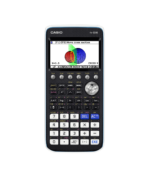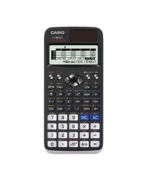Statistical Data Archives - Casio Calculators

# Statistical Data

Displaying Resources

FilterHow to Video
fx-CG50 A-Level GCSE IB
Quick Skill: Quartiles – Using Summary Values

How to extract the quartile values of single-variable data found using the Stati...How to Video
fx-CG50 A-Level GCSE IB
Quick Skill: Statistics – Using Summary Values

How to extract the summary statistic values of single-variable data found using ...How to Video
fx-CG50 A-Level GCSE IB
Statistics – Managing Lists

How to enter and manipulate data in Lists. Set frequency tables. Sort entries a...How to Video
fx-CG50 A-Level GCSE IB
Single Variable Statistics

How to calculate single variable statistics such as mean, standard deviation and...How to Video
fx-CG50 A-Level GCSE IB
Histograms – Graphing

How to plot a histogram and compare two different histograms....How to Video
fx-CG50 A-Level GCSE IB
Boxplots – Plotting and Values

How to plot a box plot and calculate single variable statistics such as mean, st...How to Video
fx-CG50 A-Level GCSE IB
Bivariate Data and Regression – Scatter Diagrams

How to plot a scatter diagram. Use linear regression to calculate regression sta...How to Video
A-Level GCSE
Grouped Data Statistics

How to enter grouped data in a frequency table and calculate the mean, quartiles...How to Video
GCSE
Frequency Tables

How to create a grouped data frequency table and calculate summary statistics su...How to Leaflet
fx-991EX A-Level GCSE
Statistics Quick Start fx-991EX

How to calculate single variable and bivariate statistics such as mean, standard...How to Leaflet
fx-CG50
Statistics – Managing Lists (Worksheet)

Worksheet to accompany the video on how to enter and manipulate data in Lists. S...How to Leaflet
fx-CG50 IB A-Level GCSE
Single Variable Statistics (Worksheet)

Worksheet to accompany the video on how to calculate single variable statistics ...How to Leaflet
fx-CG50 GCSE IB A-Level
Histograms – Graphing (Worksheet)

Worksheet to accompany the video on how to plot a histogram and compare two diff...How to Leaflet
fx-CG50 A-Level GCSE IB
Boxplots – Plotting and Values (Worksheet)

Worksheet to accompany the video on how to plot a box plot and calculate single ...How to Leaflet
fx-CG50 A-Level GCSE IB
Bivariate Data and Regression – Scatter Diagrams (Worksheet)

Worksheet to accompany the video on how to plot a scatter diagram. Use linear re...How to Leaflet
fx-CG50 A-Level GCSE IB
Statistical Charts Quick Start fx-CG50

How to create basic statistical charts on the fx-CG50. Includes pie-charts, bar-...How to Leaflet
A-Level GCSE IB
Statistics Quick Start fx-9860GII

How to create basic statistical charts on the fx-9860GII. Includes histograms an...